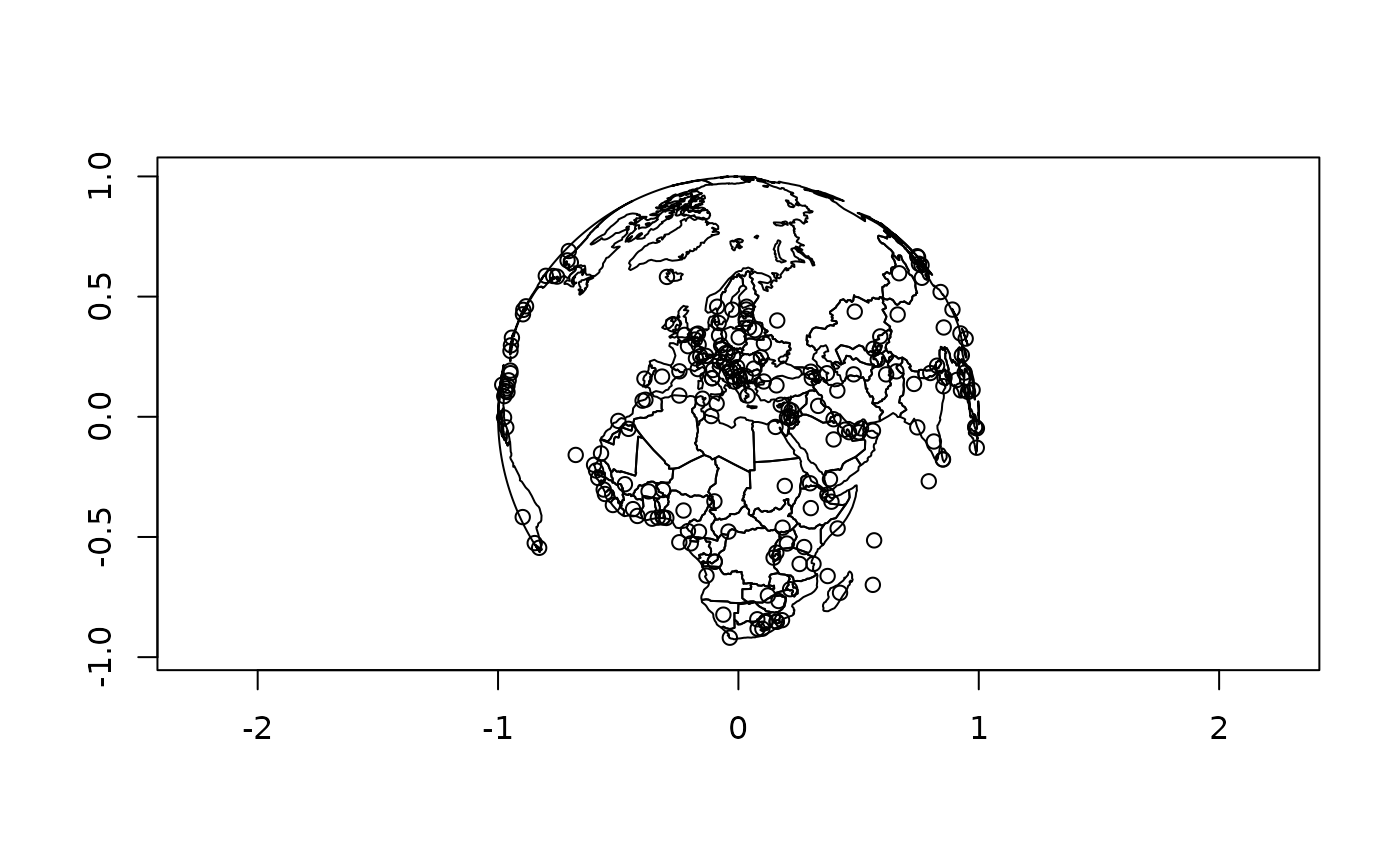Plot S2 Geographies

## Usage

s2_plot(
x,
...,
asp = 1,
xlab = "",
ylab = "",
rule = "evenodd",
plot_hemisphere = FALSE,
simplify = TRUE,
centre = NULL
)

## Arguments

x

A wkb() or wkt()

...

Passed to plotting functions for features: graphics::points() for point and multipoint geometries, graphics::lines() for linestring and multilinestring geometries, and graphics::polypath() for polygon and multipolygon geometries.

asp, xlab, ylab

Passed to graphics::plot()

rule

The rule to use for filling polygons (see graphics::polypath())

Should a new plot be created, or should handleable be added to the existing plot?

plot_hemisphere

Plot the outline of the earth

simplify

Use FALSE to skip the simplification step

centre

The longitude/latitude point of the centre of the orthographic projection

## Value

The input, invisibly

## Examples

s2_plot(s2_data_countries())